Test: Heat Exchanger Level - 1

# Test: Heat Exchanger Level - 1

Test Description

## 10 Questions MCQ Test Heat Transfer | Test: Heat Exchanger Level - 1

Test: Heat Exchanger Level - 1 for Chemical Engineering 2022 is part of Heat Transfer preparation. The Test: Heat Exchanger Level - 1 questions and answers have been prepared according to the Chemical Engineering exam syllabus.The Test: Heat Exchanger Level - 1 MCQs are made for Chemical Engineering 2022 Exam. Find important definitions, questions, notes, meanings, examples, exercises, MCQs and online tests for Test: Heat Exchanger Level - 1 below.
Solutions of Test: Heat Exchanger Level - 1 questions in English are available as part of our Heat Transfer for Chemical Engineering & Test: Heat Exchanger Level - 1 solutions in Hindi for Heat Transfer course. Download more important topics, notes, lectures and mock test series for Chemical Engineering Exam by signing up for free. Attempt Test: Heat Exchanger Level - 1 | 10 questions in 25 minutes | Mock test for Chemical Engineering preparation | Free important questions MCQ to study Heat Transfer for Chemical Engineering Exam | Download free PDF with solutions
 1 Crore+ students have signed up on EduRev. Have you?
Test: Heat Exchanger Level - 1 - Question 1

### For a heat exchanger the inlet and outlet temperatures of hot and cold fluid areThe type of heat exchanger isTh1 = 150oC Tc1 = 20oCTh2 = 70oC Tc2 = 80oC

Detailed Solution for Test: Heat Exchanger Level - 1 - Question 1 From the given condition of temperature, we see that exit temperature of cold fluid is greater than exit temperature of hot fluid. This is possible only in case of counter flow heat exchanger.

Test: Heat Exchanger Level - 1 - Question 2

### It is given that for a heat exchangerTc1 and Tc2 are the temperatures of cold fluid at entry and exit respectively and are the temperatures of hot fluid at entry and exit point. If cold fluid has lower heat capacity rate as compared to hot fluid then effectiveness of the heat exchanger is given by

Detailed Solution for Test: Heat Exchanger Level - 1 - Question 2

Effectiveness, ε = Qact/Qmax

Qact = mhcph(Th1 - Th2) = mccpc (Tc2 - Tc1)

Qmax = (mcp)small(Th1 - Tc1)

Here (mcp)c < (mcp )h

∴ ε (mcp)c (Tc2 - Tc1)/(mcp)c(Th1 - Th2)

⇒ ε = (Tc2 - Tc1)/(Th1 - Tc1)

Test: Heat Exchanger Level - 1 - Question 3

### Which one of the following heat exchanger gives parallel straight line pattern of temperature distribution for both cold and hot fluid

Detailed Solution for Test: Heat Exchanger Level - 1 - Question 3 For a parallel flow heat exchanger, the temperature profile is always converging except when both fluids are changing phase. But in case of counter flow heat exchanger i

mHcH = mccc

Then

THi - THo = Tco - Tci

∴ THi - Tco = THo - Tci

i.e. θ(x = 0) = θ(x = l)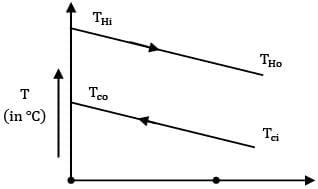x = 0 x = l x

Test: Heat Exchanger Level - 1 - Question 4

In case of boiler in a steam power plant which of the following configurations gives maximum logarithmic mean temperature difference for given values of heat capacity ratios, inlet and outlet temperatures, over all heat transfer coefficient and heat exchange area

Detailed Solution for Test: Heat Exchanger Level - 1 - Question 4 In a boiler, water changes phase to steam by absorbing heat from hot fluid gasses formed by combustion of coal. During this process the temperature of water remains constant. As a result LMTD of heat exchanger is same in both configurations whether parallel or counter flow.

Test: Heat Exchanger Level - 1 - Question 5

Consider the following statements The flow configuration in a heat exchanger whether counter flow or otherwise, will not matter if 1. a liquid is evaporating 2. a vapour is condensing 3. mass flow rate of one of the fluids is far greater Which of these statements correct?

Detailed Solution for Test: Heat Exchanger Level - 1 - Question 5 The configuration of the heat exchanger does not matter if one of the fluids is changing phase or capacity rate of one fluid is far greater than capacity rate of the other fluid.

Test: Heat Exchanger Level - 1 - Question 6

Hot gases enter a heat exchanger at and leave at . The cold air enters at and leaves at . The capacity ratio of the heat exchanger will be

Detailed Solution for Test: Heat Exchanger Level - 1 - Question 6 from energy balance we have,

Ch(Thi - The) = Cc(Tce - Tci)

⇒ Ch/Cc = (140-40)/(200-150) = 100/50 = 2

Or

Cc/Ch = 0.5

Test: Heat Exchanger Level - 1 - Question 7

What does NTU indicate?

Detailed Solution for Test: Heat Exchanger Level - 1 - Question 7

NTU = UA/Cmin

∵ NTU ∝ A, it indicates size of heat exchanger

Test: Heat Exchanger Level - 1 - Question 8

For a balanced counter flow heat exchanger (i.e. mh ch = mc cc), NTU is equal to 1.0. What is the effectiveness of the heat exchanger?

Detailed Solution for Test: Heat Exchanger Level - 1 - Question 8 For a balanced counter flow heat exchanger effectiveness is given as

ε = NTU/1+NTU = 1/1+1

⇒ ε = 1/2

⇒ ε = 0.5

Test: Heat Exchanger Level - 1 - Question 9

Which of the following temperature profiles represents a counter flow exchanger with heat capacity rate of hot fluid (CH) greater than heat capacity rate of cold fluid (CC)

(A)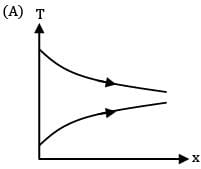(B)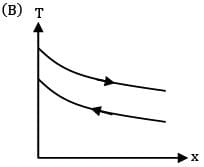(C)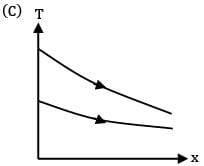(D)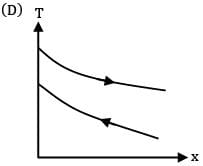Detailed Solution for Test: Heat Exchanger Level - 1 - Question 9 For a counter flow heat exchanges, the two fluids flow in opposite directions. Also by steady state flow energy equation

CH(THi - THo) = Cc(Tco - Tci)

Given CH > CC

∴ THi -THo < />co -TCi

∴ THi -TCo < />Ho -TCi

∴ ፀ( x = 0) < ፀ(="" x="" />

Thus correct profile is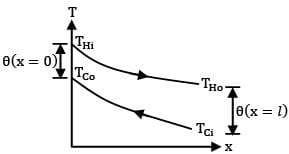Test: Heat Exchanger Level - 1 - Question 10

Match List-I with List-II and select the correct answer using the code given below the lists

List-I

a. Number of transfer units

b. Periodic flow heat exchangers

c. Phase change

d. Deposition on heat exchanger surface

List-II

1. Regenerators

2. Fouling factor

3. A measure of heat exchanger size

4. Condensers

Codes: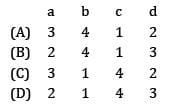## Heat Transfer

58 videos|70 docs|85 tests
 Use Code STAYHOME200 and get INR 200 additional OFF Use Coupon Code
Information about Test: Heat Exchanger Level - 1 Page
In this test you can find the Exam questions for Test: Heat Exchanger Level - 1 solved & explained in the simplest way possible. Besides giving Questions and answers for Test: Heat Exchanger Level - 1, EduRev gives you an ample number of Online tests for practice

## Heat Transfer

58 videos|70 docs|85 tests